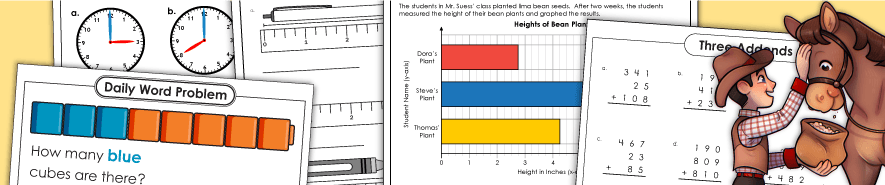# Math Skills WorksheetsUse these worksheets to practice one, two, three, four, and five-digit adding. There are also worksheets on adding ten and multiples of ten.

Counting

This page has printable worksheets to help students learn to count up to 10, 20, 30, and beyond. It also includes worksheets on skip counting and counting backwards.

Daily Math Review (Math Buzz)

This page links to daily math review worksheets by grade. Each level includes 150 worksheets, so students can make Math Buzz part of their daily school routine.

Daily Word Problems

Students in kindergarten through 4th grade can solve a different word problem every day with these worksheets.

Division (Basic)

Division (Long Division)

This page has links to lots of worksheets and activities on long division. Concepts include division with 2, 3, and 4-digit dividends, as well as division with money and decimals.

Fractions

Print out fraction strips, fraction circles, and fraction worksheets. Concepts covered include reciprocal fractions, comparing and ordering fractions, and adding, subtracting, multiplying, and dividing fractions.

Geometry

These geometry worksheets cover topics such as area, perimeter, lines, rays, line segments, and more!

Graphing

Check out our collection of printable graphing worksheets, featuring bar graphs, line graphs, circle graphs, and more.

Measurement

These worksheets help students learn about many types of measurement, from linear measurements (inches, feet, yards, etc.) to volume, weight, and temperature measurements.

Multiplication (Basic)

In this section of our site, you'll find basic facts multiplication worksheets. You can also print multiplication tables, flashcards, task cards, and games. Links to more advanced, multi-digit multiplication pages are also provided.

Multiplication (Multi-Digit)

Use the worksheets on this page to reinforce skills related to multiplying by 2, 3, and 4-digit numbers. Worksheets on lattice multiplication, multiplying money, multiplying decimals, and multiplying fractions are also included.

Place Value

With these worksheets, students can practice place value up to 12 digits. They can also practice decimal and money place value with tenths, hundredths, and thousandths.

Rounding

Use these worksheets to help students learn to round numbers to the nearest ten, hundred, or thousand. This page also includes decimal and money rounding worksheets.

Subtraction

These printable worksheets help students practice subtraction with and without borrowing.

Time

These worksheets will give students lots of practice telling time to the nearest fifteen minutes, nearest five minutes, and nearest minute.